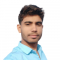## Half Subtractor Using NAND GatesUpdated on 26-Dec-2022 18:14:43

4K+ Views

In digital electronics, a subtractor is a combinational logic circuit that performs the subtraction of two binary numbers. However, the subtraction of binary number can be performed using adder circuits by taking 1’s or 2’s compliments. But, we may also realize a dedicate circuit to perform the subtraction of two binary numbers. In the subtraction of two binary numbers, each subtrahend bit of the number is subtracted from its corresponding significant minuend bit to form a difference bit. During the subtraction, if the minuend bit is smaller than the subtrahend bit, then a 1 is borrowed from the next position. ... Read More

## Half Subtractor in Digital ElectronicsUpdated on 26-Dec-2022 18:12:00

6K+ Views

In digital electronics, a subtractor is a combinational logic circuit that can perform the subtraction of two number (binary numbers) and produce the difference between them. It is a combinational circuit that means its output depends on its present inputs only. Although, in practice, the subtraction of two binary number is accomplished by taking the 1's or 2's compliment of the subtrahend and adding it to the minuend. In this way, the subtraction operation of binary numbers can be converted into simple addition operation which makes hardware construction simple and less expensive. There are two types of subtractors namely, Half ... Read More